# Minerva: Solving Quantitative Reasoning Problems with Language Models

Language models have demonstrated remarkable performance on a variety of natural language tasks — indeed, a general lesson from many works, including BERT, GPT-3, Gopher, and PaLM, has been that neural networks trained on diverse data at large scale in an unsupervised way can perform well on a variety of tasks.

Quantitative reasoning is one area in which language models still fall far short of human-level performance. Solving mathematical and scientific questions requires a combination of skills, including correctly parsing a question with natural language and mathematical notation, recalling relevant formulas and constants, and generating step-by-step solutions involving numerical calculations and symbolic manipulation. Due to these challenges, it is often believed that solving quantitative reasoning problems using machine learning will require significant advancements in model architecture and training techniques, granting models access to external tools such as Python interpreters, or possibly a more profound paradigm shift.

In “Solving Quantitative Reasoning Problems With Language Models”, we present Minerva, a language model capable of solving mathematical and scientific questions using step-by-step reasoning. We show that by focusing on collecting training data that is relevant for quantitative reasoning problems, training models at scale, and employing best-in-class inference techniques, we achieve significant performance gains on a variety of difficult quantitative reasoning tasks. Minerva solves such problems by generating solutions that include numerical calculations and symbolic manipulation without relying on external tools such as a calculator. The model parses and answers mathematical questions using a mix of natural language and mathematical notation. Minerva combines several techniques, including few-shot prompting, chain of thought or scratchpad prompting, and majority voting, to achieve state-of-the-art performance on STEM reasoning tasks. You can explore Minerva’s output with our interactive sample explorer!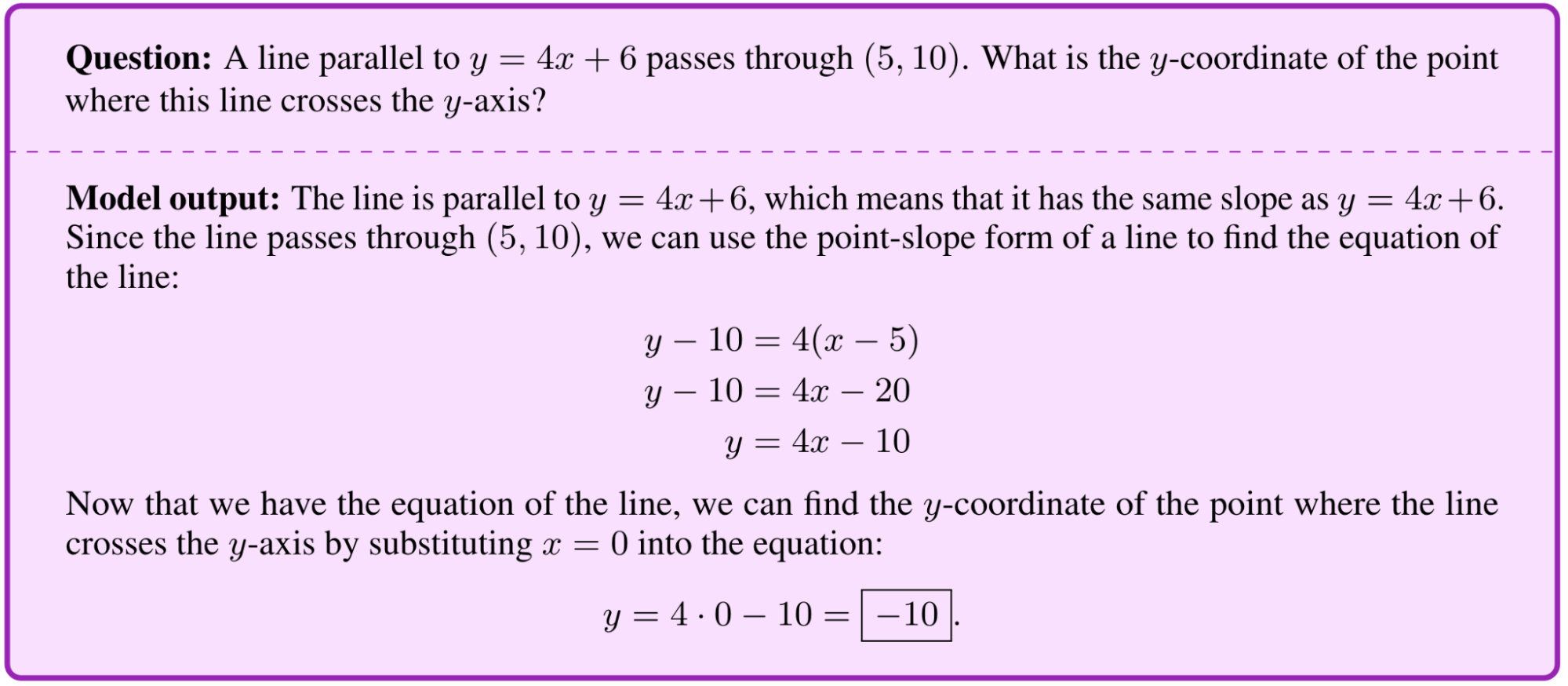Solving a multi-step problem: A question from the MATH dataset and Minerva’s solution. The model writes down a line equation, simplifies it, substitutes a variable, and solves for y.

## A Model Built for Multi-step Quantitative Reasoning

To promote quantitative reasoning, Minerva builds on the Pathways Language Model (PaLM), with further training on a 118GB dataset of scientific papers from the arXiv preprint server and web pages that contain mathematical expressions using LaTeX, MathJax, or other mathematical typesetting formats. Standard text cleaning procedures often remove symbols and formatting that are essential to the semantic meaning of mathematical expressions. By maintaining this information in the training data, the model learns to converse using standard mathematical notation.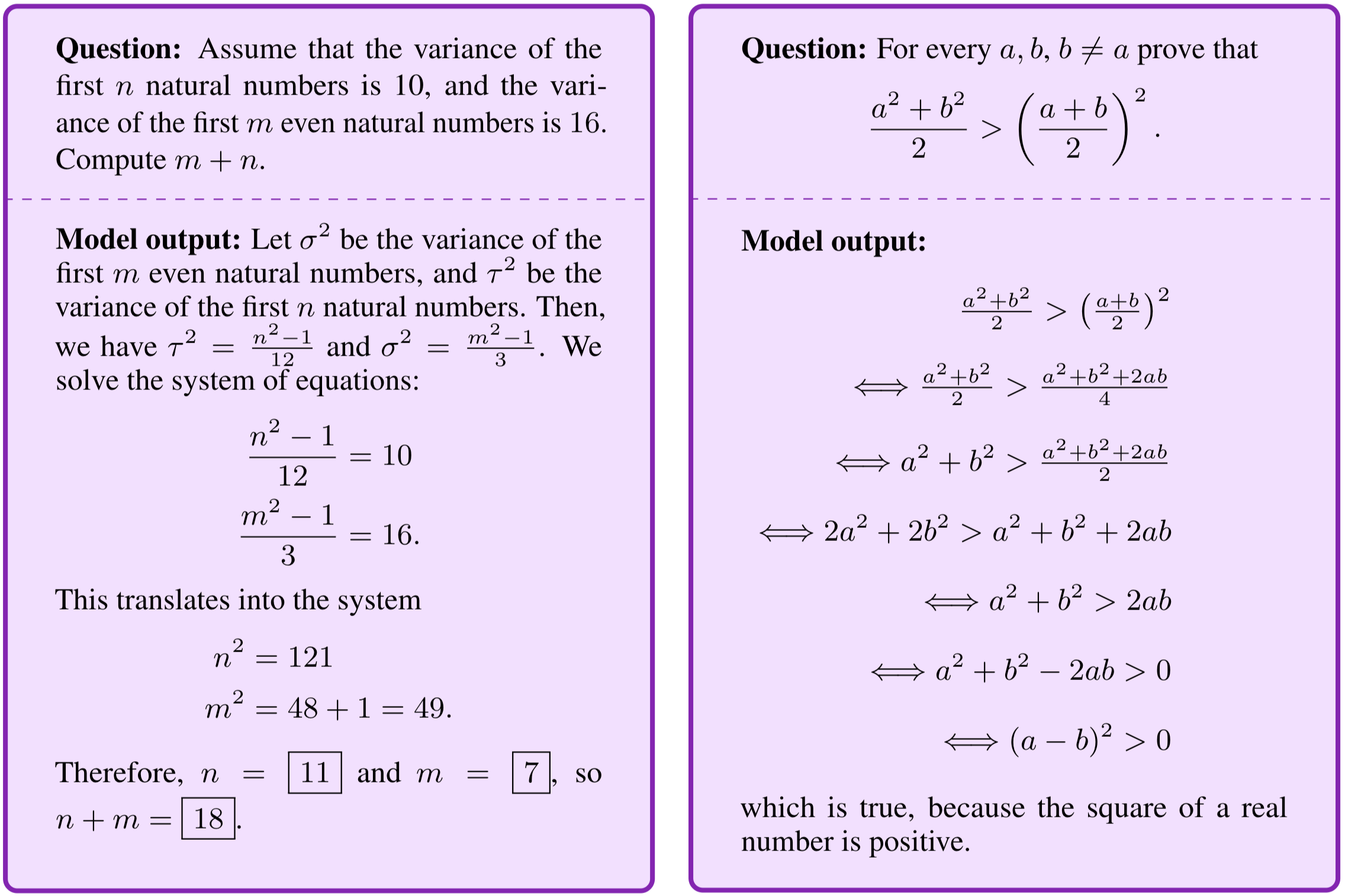Example questions from the Joint Entrance Examination Main Math 2020 exam taken each year by almost 2M Indian high-school students intended to study engineering and similar fields (left), and the National Math Exam in Poland (May 2022) taken by approximately 270K high-school students every year (right).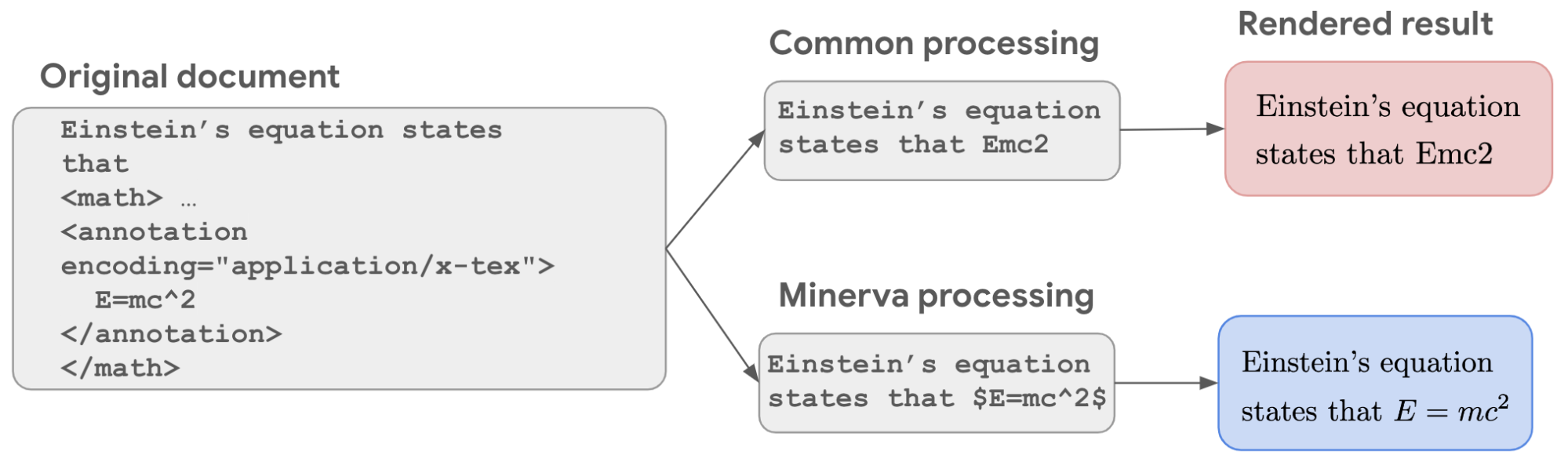A dataset for quantitative reasoning: Careful data processing preserves mathematical information, allowing the model to learn mathematics at a higher level.

Minerva also incorporates recent prompting and evaluation techniques to better solve mathematical questions. These include chain of thought or scratchpad prompting — where Minerva is prompted with several step-by-step solutions to existing questions before being presented with a new question — and majority voting. Like most language models, Minerva assigns probabilities to different possible outputs. When answering a question, rather than taking the single solution Minerva scores as most likely, multiple solutions are generated by sampling stochastically from all possible outputs. These solutions are different (e.g., the steps are not identical), but often arrive at the same final answer. Minerva uses majority voting on these sampled solutions, taking the most common result as the conclusive final answer.Majority voting: Minerva generates multiple solutions to each question and chooses the most common answer as the solution, improving performance significantly.

## Evaluation on STEM Benchmarks

To test Minerva’s quantitative reasoning abilities we evaluated the model on STEM benchmarks ranging in difficulty from grade school level problems to graduate level coursework.

• MATH: High school math competition level problems
• MMLU-STEM: A subset of the Massive Multitask Language Understanding benchmark focused on STEM, covering topics such as engineering, chemistry, math, and physics at high school and college level.
• GSM8k: Grade school level math problems involving basic arithmetic operations that should all be solvable by a talented middle school student.

We also evaluated Minerva on OCWCourses, a collection of college and graduate level problems covering a variety of STEM topics such as solid state chemistry, astronomy, differential equations, and special relativity that we collected from MIT OpenCourseWare.

In all cases, Minerva obtains state-of-the-art results, sometimes by a wide margin.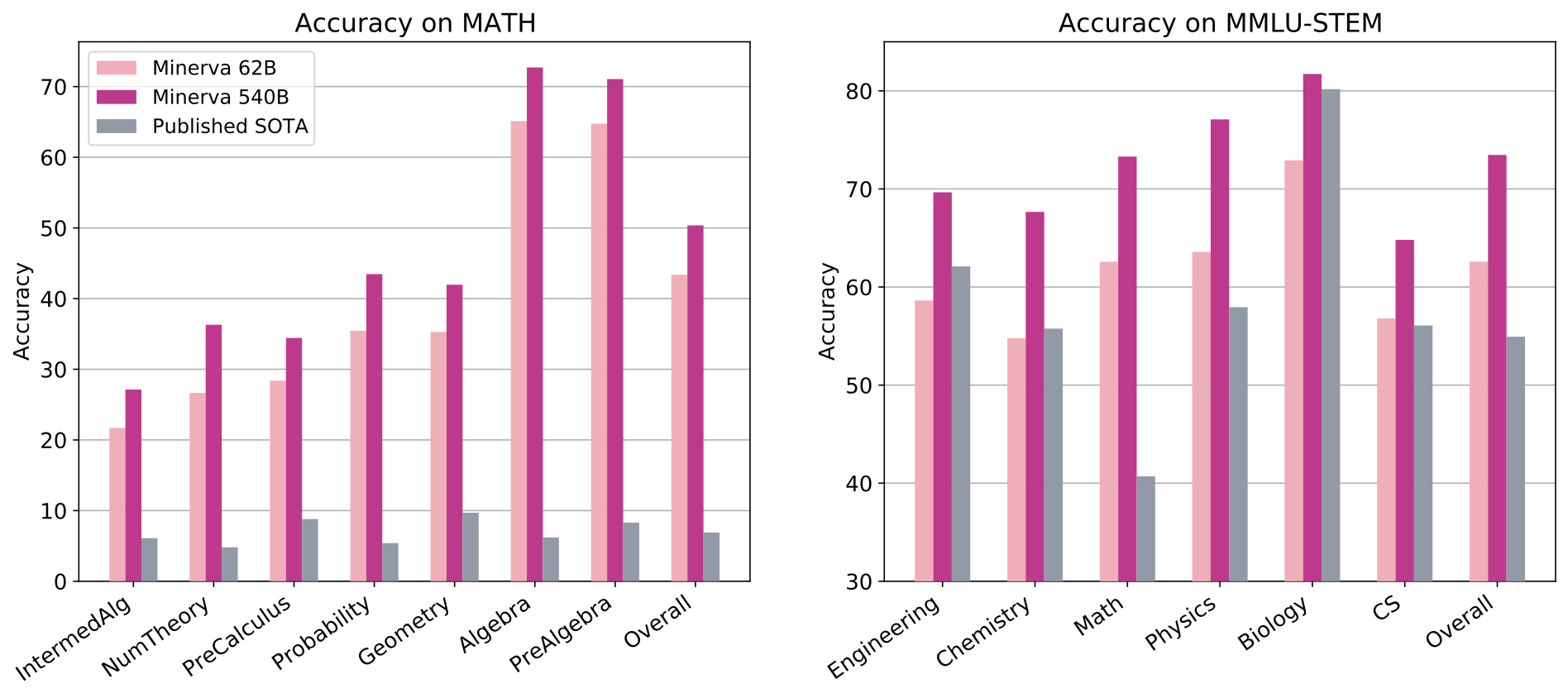Evaluation results on MATH and MMLU-STEM, which include high school and college level questions covering a range of STEM topics.

 Model MATH MMLU-STEM OCWCourses GSM8k Minerva 50.3% 75% 30.8% 78.5% Published state of the art 6.9% 55% - 74.4%
 Minerva 540B significantly improves state-of-the-art performance on STEM evaluation datasets.

## What Minerva Gets Wrong

Minerva still makes its fair share of mistakes. To better identify areas where the model can be improved, we analyzed a sample of questions the model gets wrong, and found that most mistakes are easily interpretable. About half are calculation mistakes, and the other half are reasoning errors, where the solution steps do not follow a logical chain of thought.

It is also possible for the model to arrive at a correct final answer but with faulty reasoning. We call such cases “false positives”, as they erroneously count toward a model’s overall performance score. In our analysis, we find that the rate of false positives is relatively low (Minerva 62B produces less than 8% false positives on MATH).

Below are a couple of example mistakes the model makes.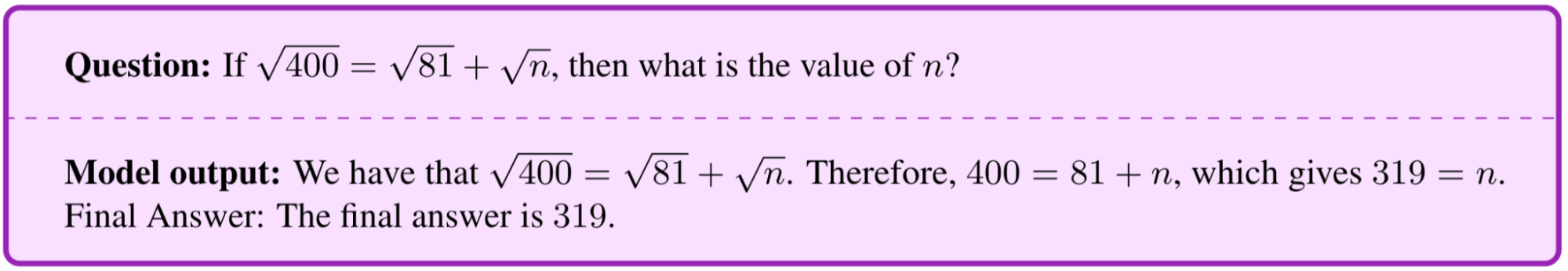Calculation mistake: The model incorrectly cancels the square root on both sides of the equation.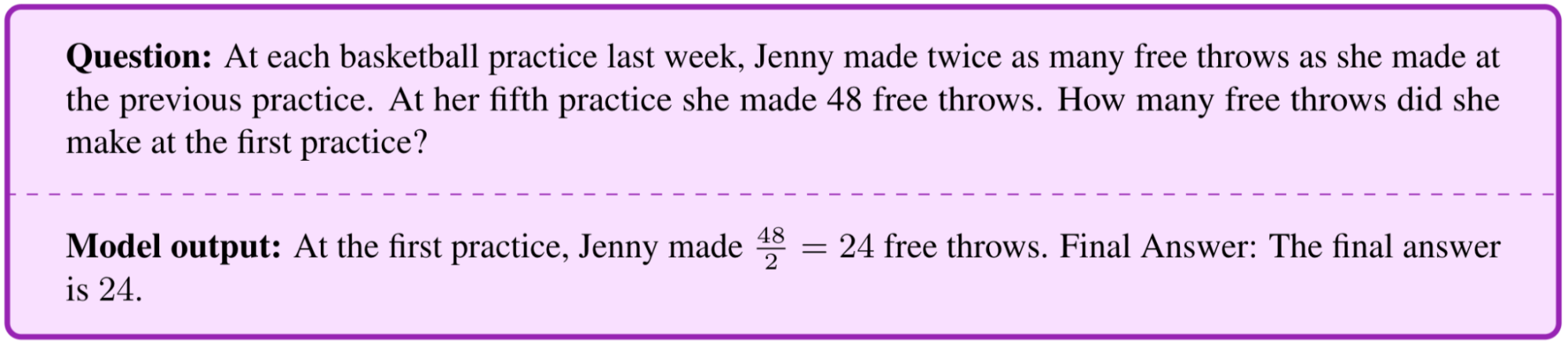Reasoning mistake: The model computes the number of free throws at the fourth practice, but then uses this number as the final answer for the first practice.

## Limitations

Our approach to quantitative reasoning is not grounded in formal mathematics. Minerva parses questions and generates answers using a mix of natural language and LaTeX mathematical expressions, with no explicit underlying mathematical structure. This approach has an important limitation, in that the model’s answers cannot be automatically verified. Even when the final answer is known and can be verified, the model can arrive at a correct final answer using incorrect reasoning steps, which cannot be automatically detected. This limitation is not present in formal methods for theorem proving (e.g., see Coq, Isabelle, HOL, Lean, Metamath, and Mizar). On the other hand, an advantage of the informal approach is that it can be applied to a highly diverse set of problems which may not lend themselves to formalization.

## Future Directions

While machine learning models have become impressive tools in many scientific disciplines, they are often narrowly scoped to solve specific tasks. We hope that general models capable of solving quantitative reasoning problems will help push the frontiers of science and education. Models capable of quantitative reasoning have many potential applications, including serving as useful aids for researchers, and enabling new learning opportunities for students. We present Minerva as a small step in this direction. To see more samples from Minerva, such as the one below, please visit the interactive sample explorer!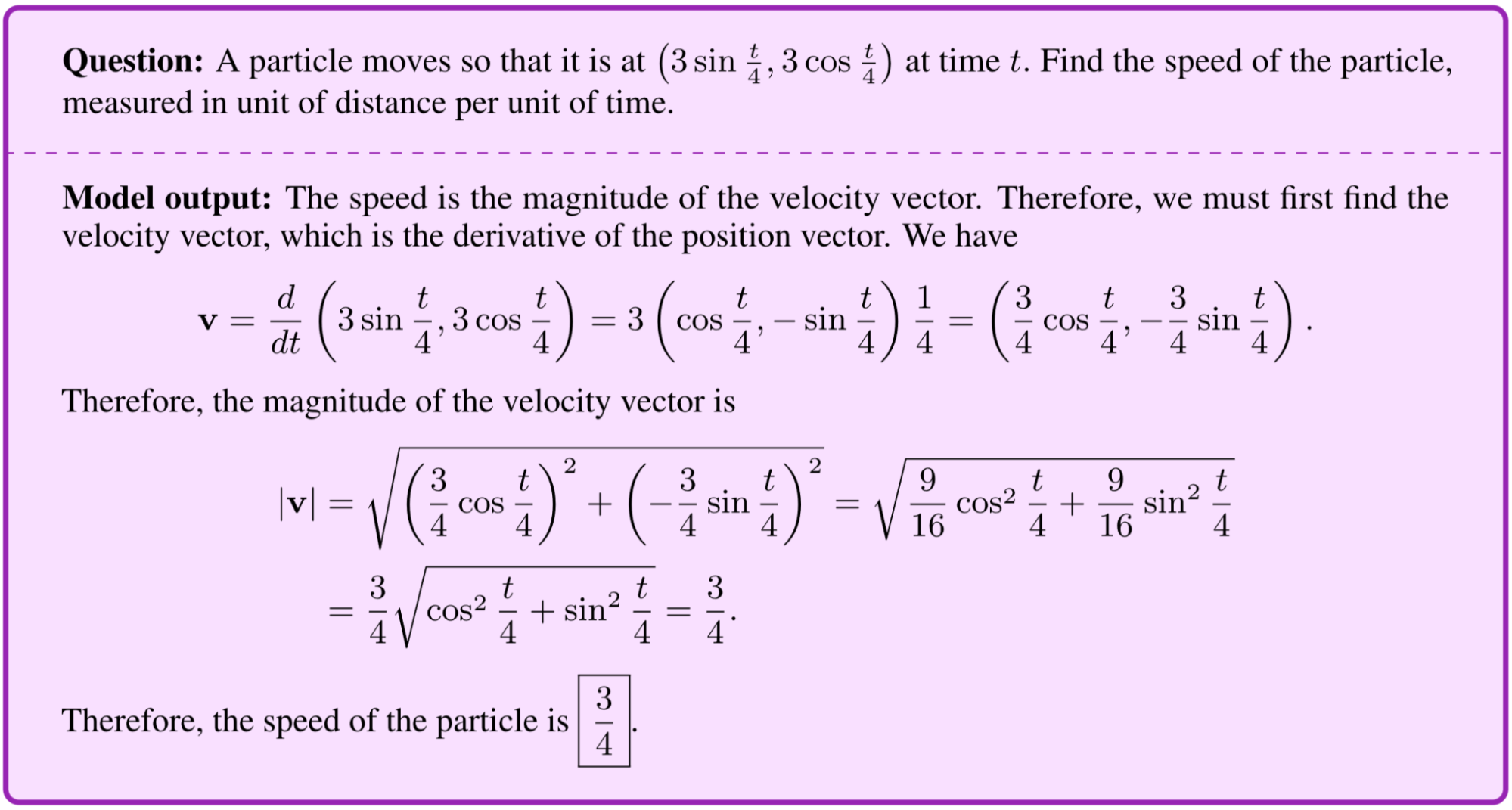Solving a problem using calculus and trigonometry: A question from the MATH dataset asking for the speed of a particle in circular motion. Minerva finds a correct step-by-step solution. In the process, Minerva computes a time derivative and applies a trigonometric identity.

## Acknowledgements

Minerva was a collaborative effort that spanned multiple teams in Google Research. We would like to thank our coauthors Aitor Lewkowycz, Ambrose Slone, Anders Andreassen, Behnam Neyshabur, Cem Anil, David Dohan, Henryk Michalewski, Imanol Schlag, Theo Gutman-Solo, Vedant Misra, Vinay Ramasesh, and Yuhuai Wu, as well as our collaborators Eric Zelikman and Yasaman Razeghi. Minerva builds upon the work of many others at Google, and we would like to thank the PaLM team, the T5X team, the Flaxformer team, and the JAX team for their efforts. We thank Tom Small for designing the animation in this post. We would also like to especially thank Vedant Misra for developing the Minerva sample explorer.